## Using an Extended Kalman Filter for Object Tracking in Simulink

This tutorial presents an example of how to implement an Extended Kalman filter in Simulink. If you are unfamiliar with Simulink then look here for some generic Simulink tutorials discussing how to build and execute simple models. If you are unfamiliar with the mathematics behind the Kalman Filter or the Extended Kalman Filter then see the linked tutorials.

One of the earliest applications of the Extended Kalman Filter was to solve the problem of tracking flying objects. The basic problem is shown in Figure 1.Figure 1: Relationship between displacements and range-bearing.

At each point in time the object being tracked has a given range and bearing from the observer. Often the observer is considered the location of a radar dish tracking the object. The range and bearing are generated from displacements (i.e. distances from the observer) in both the x and y directions. (This tutorial does not consider the altitude of the object.)

The tracking problem involves estimating not only the x and y displacements of the object but also its x and y velocities. These four states must be estimated given only noisy measurements of range and bearing. Given that the displacements and velocities are non-linearly related to the range and bearing this is an ideal problem to solve using an Extended Kalman Filter.

The Extended Kalman Filter algorithm requires the calculation of Jacobian matrices for the state and measurement equations. These take the following forms. Over a small period of time the displacement can be considered to change according to the first order approximation,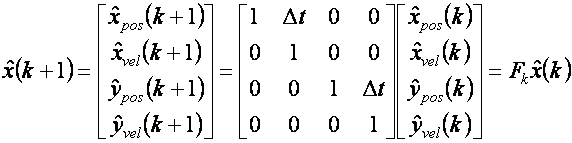The above equation says that over a small period of time the position changes by Δt times the velocity (in both the x and y directions) and that the velocity remains constant (in both the x and y directions). Fk is the required Jacobian matrix.

The measurement update equation is slightly more complex – relying on the differentiation of a trigonometric identity. The range and bearing are related to the x and y displacements by the equations,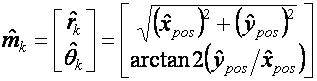(Note that the sample time subscript k has been dropped from the state vector to avoid cluttering the equations.) Hence the Jacobian for the measurement equations is given by,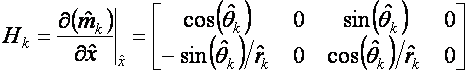A Simulink model that implements the basic tracking problem discussed above and which uses an Extended Kalman Filter to estimate the object's trajectory is shown in Figure 2.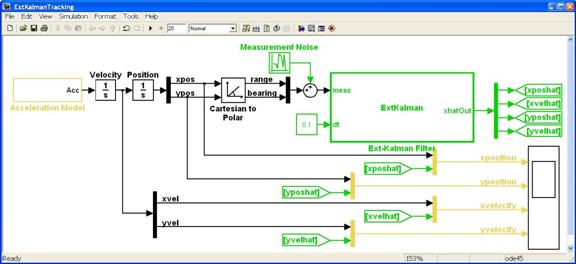Figure 2: Simulink Model for Tracking a Flying Object using an Extended Kalman Filter.

A zip file containing the model of Figure 2 may be downloaded here.

There are two main parts to this model: firstly the blocks that model the actual trajectory of the object being tracked; and secondly the Extended Kalman Filter used to estimate the trajectory of the object from measured data. Each of these is described in more detail below.

#### The Actual Trajectory

The blocks that are coloured black are used to model the actual trajectory of an object flying in 2-dimensional space. Ultimately the properties being measured are the range and bearing. These are calculated from the x and y displacements, which are generated by integrating velocities, which in turn are generated by integrating accelerations.

The accelerations are generated by the acceleration model shown in Figure 3. A simpler model could use either a constant velocity (subject to random perturbations) or a constant acceleration (subject to random perturbations). This model assumes serially correlated random accelerations. This corresponds to an object that is manoeuvering with a velocity that cannot change too quickly. (The gain in the first order filter determines the rate at which the velocity is allowed to change.)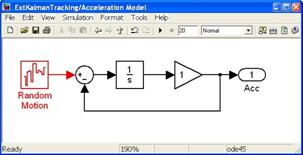Figure 3: Acceleration Model.

In this example the object is assumed to start in the north-west and be travelling due east at 100 m/s. This corresponds to the settings given in the following table,

Quantity Value
Initial x position -1000 m
Initial y position -1000 m
Initial x velocity 100 m/s
Initial y velocity 0 m/s
x displacement noise power 10
y displacement noise power 0.1

#### The Extended Kalman Filter

The Extended Kalman Filter uses a predictor-corrector algorithm to estimate unmeasured states of a discrete process. A discussion of the mathematics behind the Extended Kalman Filter may be found in this tutorial.

For the tracking problem under consideration the measured data is the object's actual range and bearing corrupted with zero-mean Gaussian noise and sampled at 0.1s intervals. The range noise has a variance of 50 while the bearing noise has a variance of 0.005.

The Extended Kalman Filter itself has been implemented using an Embedded MATLAB Function block. The block is discrete with a sample time of 0.1 seconds. The code for the block is shown below.

Note that the filter has deliberately been initialized with erroneous data to reflect that the actual trajectory is not known in advance.

```function xhatOut = ExtKalman(meas,dt)
% This Embedded MATLAB Function implements an extended Kalman filter used
% for object tracking.
%
% The states of the process are given by
% x = [x_position; x_velocity; y_position; y_velocity];
%
% and the measurements are given by
% y = [range; bearing]
%
% where
% range = sqrt(x_position^2 + y_position^2)
% bearing = atan2(y_position/x_position)

% Author: Phil Goddard (phil@goddardconsulting.ca)
% Date: Q2, 2011.

% Define storage for the variables that need to persist
% between time periods.
persistent P xhat Q R
if isempty(P)
% First time through the code so do some initialization
xhat = [-900;80;950;20];
P = zeros(4,4);
Q = diag([0 .1 0 .1]);
R = diag([50^2 0.005^2]);
end
% Calculate the Jacobians for the state and measurement equations
F = [1 dt 0 0;0 1 0 0;0 0 1 dt;0 0 0 1];
rangeHat = sqrt(xhat(1)^2+xhat(3)^2);
bearingHat = atan2(xhat(3),xhat(1));
yhat = [rangeHat; bearingHat];
H = [cos(bearingHat) 0 sin(bearingHat) 0;
-sin(bearingHat)/rangeHat 0 cos(bearingHat)/rangeHat 0];
% Propogate the state and covariance matrices
xhat = F*xhat;
P = F*P*F' + Q;
% Calculate the Kalman gain
K = P*H'/(H*P*H' + R);
% Calculate the measurement residual
resid = meas - yhat;
% Update the state and covariance estimates
xhat = xhat + K*resid;
P = (eye(size(K,1))-K*H)*P;
% Post the results
xhatOut = xhat;
```

#### Simulation Results

Simulation results are shown in Figure 4. Each of the actual and estimated states are shown on a separate axis, with the actual trajectory shown in blue and the estimated trajectory shown in red.

Note again that the estimates have deliberately been initialized with non-exact values. This means that the filter takes some time to converge to an acceptable estimate. However after 10-15 seconds the estimates track the actual trajectories to a reasonable accuracy. The estimates could be further improved by tuning the noise covariance estimates before (and during) applying the filtering.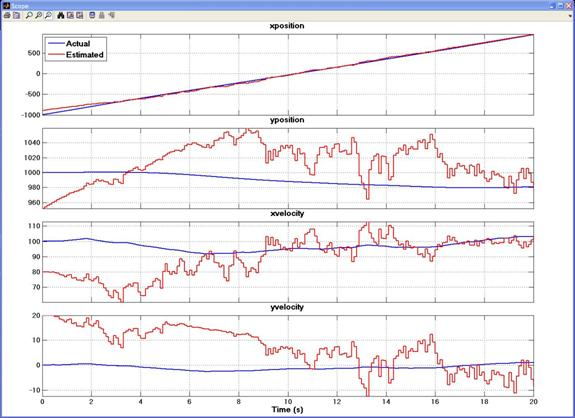Figure 4: Actual and Estimated Positions and Velocities.

It is often useful when performing trajectory tracking to display the results on a polar plot. This is shown in Figure 5. The object is moving from the northwest directly east and ending in the northeast. Starting at a range of about 1400 metres the object comes within 1km of the observer then moves out again to a range of about 1400 metres. This confirms that the filter takes a short time to correctly estimate the object's position but then does a good job at following its trajectory.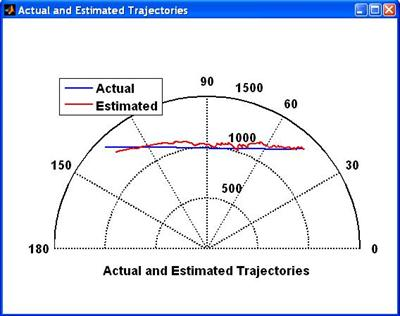Figure 5: Polar Plot of Actual and Estimated Trajectories.